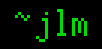## 24-Sep-2005

### Spokes

Filed under: math — jlm @ 08:27

So, can spokes help hold our Orbital (see below post) together? After all, internal tension acts mostly transverse to the centrifugal force whereas a spoke would act mostly along it. How about attaching the spoke to its opposite point on the Orbital?

Well, centripetal acceleration at a distance r from the center we already worked out to be a = r·4π²/d². If the spoke has linear density σ, then the mass of a bit of spoke Δr long would be ΔM = σΔr and have weight Δw = aΔM = r·(4π²/d²)·σΔr. So the total weight of the spoke half would be w=∫dw=∫r·(4π²/d²)·σdr = (4π²/d²)·σ∫r dr = (4π²/d²)·σ·(r²/2) = (2π²/d²)·σr²   (integrating from 0 to the Orbital’s radius).

The tension on the spoke just from its own weight is that of both halves, or T = (4π²/d²)·σr². This gives a T/σ of (4π²/d²)·r² just for the spoke to be strong enough to support itself. With d = 86400 s and r = 2×109 m, we’d need a strength of 2×1010 m²/s², which is as much as we’d need for the Orbital to support itself with internal tension anyway! Tweaking values won’t help, as in both cases the equations work out to T/σ = a·r. I think this means that diametric spokes can only be less effective than a band at holding a spinning structure (of any size) together.

What if we add a hub? Then the tension on the spoke is halved, but we have the problem of holding the hub together. We can also vary the spoke thickness, because tension is not uniform over it: Near the Orbital, it’s only carrying the support load, but at the hub it has the load from its own weight as well. With constant specific material strength S = T(r)/σ(r) and support load s, and Orbital radius R, we get the integral equation T(r) = s + ∫rR dw = s + ∫rR r·(4π²/d²)·σ(r) dr, or σ(r)·S = s – ∫Rr r·(4π²/d²)·σ(r) dr.

This gives the differential equation σ'(r)·S = -r·(4π²/d²)·σ(r) with initial condition σ(R) = s/S. At this point, I had to go and dust off my old math books. In standard form, the diff. eq. is σ'(r)+(4π²/Sd²)·rσ(r) = 0. This has solution σ(r) = (s/S)·exp(-A(r)) where A(r) = ∫Rr(4π²/Sd²)·r dr = (4π²/Sd²) ∫Rr r dr = (2π²/Sd²)·(r² – R²).

This simplifies to σ(r) = (s/S)·exp((2π²/Sd²)·(R²-r²)). At the hub r=0, so we have σ(0) = (s/S)·exp(2π²R²/Sd²). So the tension on the hub is T(0) = s·exp(2π²R²/Sd²). Kevlar has a S = 2500 MPa/(g/cm³) = 2.5×106 m²/s². So, for each pound the Orbital supports, this would place exp(4000) = 101800 lb of tension on the hub. That’s farther beyond scrith’s capabilities than scrith is beyond ours. How about that miracle nanotube cable? S = 37 GPa/(g/cm³) = 3.7×107 m²/s², so T(0)/s = exp(286) = 10124. Trying to hold it together with a hub makes the problem much harder, not easier.